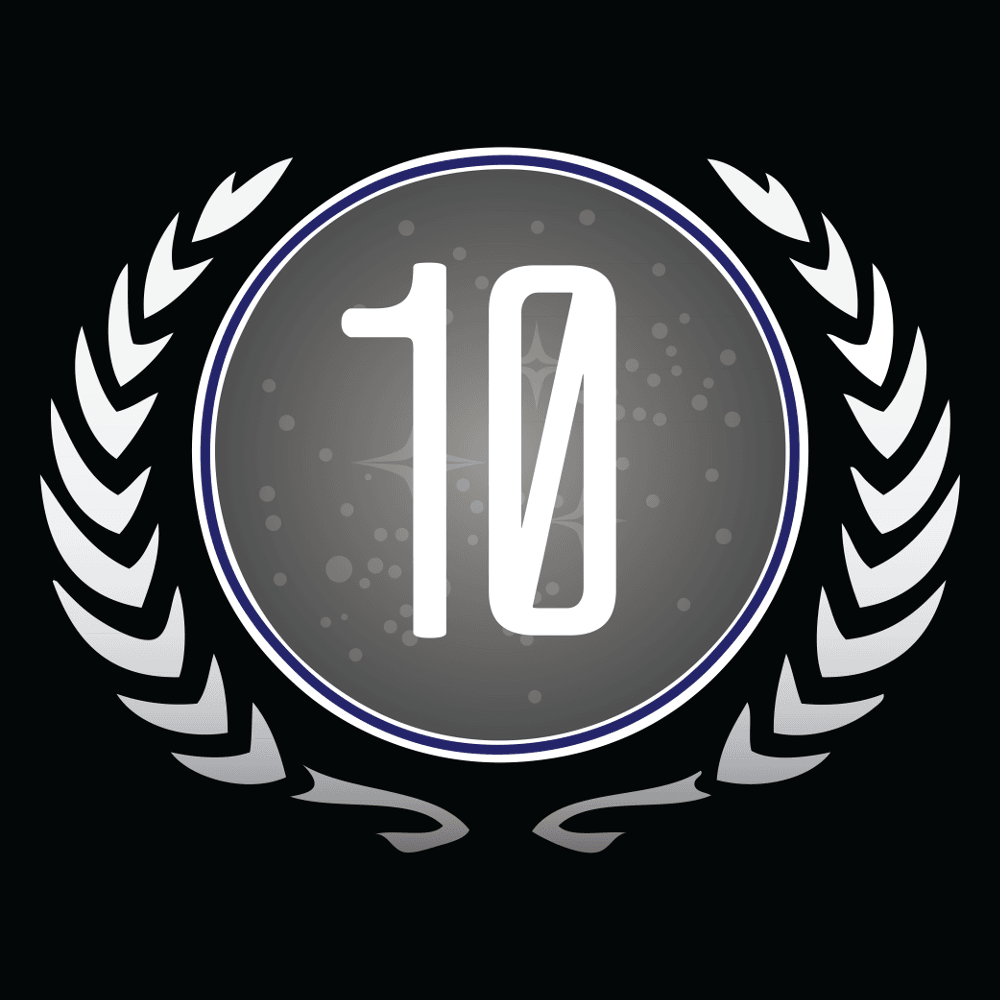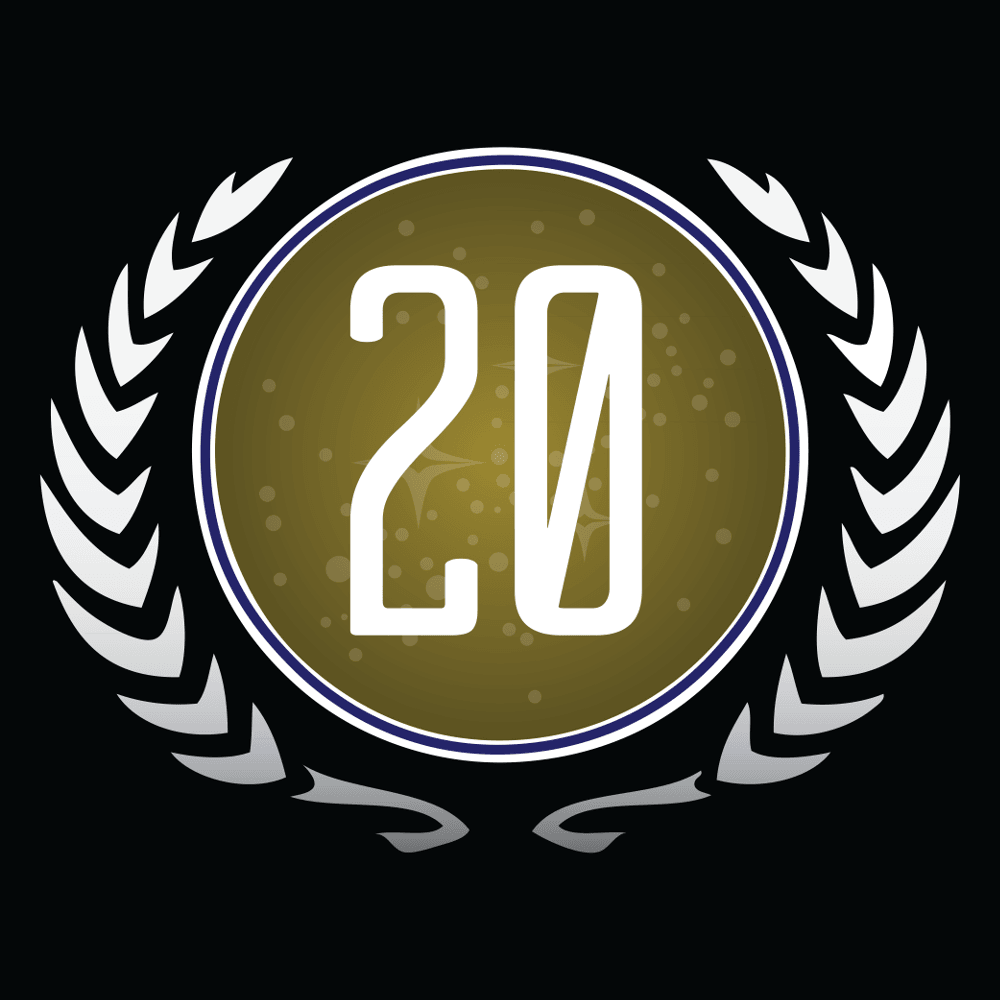## Test's AchievementsPoints for New MembersPoints for Each Forum PostPoints for Each Forum PostPoints for Each Forum PostPoints for Each Forum PostPoints for Each Forum PostPoints for Each Forum PostPoints for Each Forum PostPoints for Each Forum PostPoints for Each Forum PostPoints for Each Forum PostPoints for Each Forum PostPoints for Each Forum PostPoints for Each Forum PostPoints for Each Forum PostPoints for Each Forum PostPoints for Each Forum PostPoints for Each Forum PostPoints for Each Forum PostPoints for Each Forum PostPoints for Each Forum PostPoints for Each Forum PostPoints for Each Forum PostPoints for Each Forum PostPoints for Each Forum PostPoints for Each Forum PostPoints for Each Forum PostPoints for Each Forum PostPoints for Each Forum Post